Creating an R data package

A few weeks ago I got back into an old textbook of mine, Design and Analysis of Experiments (Dean and Voss). One of the things I love about this book is the incredible number of actual experiments used to demonstrate concepts. And all the data are available from the author’s website. My main interest in working through the book was to convert the many examples provided in SAS code into corresponding R code and to recreate the many plots. To do that meant having to download the data from the website and read it into R. Not that big of a deal, really, but I started thinking how nice it would be if the data were in an R package. Then I could just load the package and use the data at will. And I could document the data so I wouldn’t have to refer back to the book for variable definitions. And that’s what put me on the road to creating my first R data package, dvdata.

Now you’ll notice that last link went to GitHub, instead of CRAN. That’s because I asked one of the authors after I built the package if he would mind me uploading it to CRAN. Unfortunately, he did mind, because it turns out he’s working on his own R package for the next edition of the book. I was a little bummed, because I really wanted it on CRAN for the warm feeling of authenticity. But I understand. And besides the package still does what I wanted all along.

Now let’s talk about creating an R package. The very first thing you want to do is head over to Hadley Wickham’s R Packages site. He wrote a lovely book on how to create R Packages and posted it for free. And because it’s online, it almost always up-to-date. Hadley gently walks you through the process of creating an R package using his devtools package. I found it very easy to follow and I can’t recommend it enough.

What I want to do in this post is document in one place the basic steps to creating a R data package. All of these steps are in Hadley’s book, but they’re a little spread out due to the structure of the book, and because he covers a lot more than just making a simple data package.

Before you start, follow the directions under Getting Started in the Intro to Hadley’s book.

Steps to making an R data package

1. come up with a name for your package and create a package in RStudio as described here. This creates the smallest usable package. Let’s say you named your package “grrr”. On your computer you now have a directory called “grrr” which contains your package folders and files.

2. create two new directories: “data” and “data-raw” in your package directory.

3. go to the “R” directory in your package and delete the “hello.R” file.

4. Start a new R script called, perhaps, “get_data.R” and save to the “raw-data” directory. This will be the R script that reads in your data, wrangles it into shape and saves the data as an .RData file. You need to save the .RData objects into the “data” directory. The .RData objects are the data frames (or lists, or matrices, or vectors) your package will allow you to easily load. For examples, see Hadley’s R scripts in the “data-raw” directory of his babynames package.

5. When your data objects are done (i.e., the .RData files in your “data” directory) start an R script called “data.R” in the “R” directory. This is where you will compose the documentation for your data objects. Follow the directions in this section of Hadley’s book.

6. As you write your documentation, follow the The Documentation Workflow Hadley outlines in this section. Basically this involves submitting devtools::document() from the console and then previewing the documentation. Each time you submit devtools::document() Rd files are generated in the “man” directory of your package. (If you didn’t have a “man” directory, devtools creates one for you.) Do this until you are satisfied with your documentation.

7. Update the DESCRIPTION file as explained in this section. DESCRIPTION is just a text file.

8. Add ^data-raw$to .Rbuildignore file. It too is just a text file. That keeps the “data-raw” folder from being included when the package is built. 9. Build the package: Ctrl + Shift + B. Feel free to do this at any point as you’re working on your package. That about does it! If you want to submit to CRAN, then read Hadley’s Release chapter very closely and follow it to the T. After creating dvdata, I created another data package called valottery that contains historical results of Virginia lottery drawings. This one I did get uploaded to CRAN. A Note on Boxplots in R As a statistical consultant I frequently use boxplots. They're a great way to quickly visualize the distribution of a continuous measure by some grouping variable. More often than not, however, the person I'm helping doesn't regularly use boxplots (if at all) and is not sure what to make of them. Fortunately, boxplots are pretty easy to explain. The line in the middle of the box is the median. The box itself represents the middle 50% of the data. The box edges are the 25th and 75th percentiles. But what about the whiskers? That seems to be the part that trips people up. Before we go further, let's make some boxplots in R: set.seed(9) y <- rnorm(n = 100, mean = rep(c(22:25),each=25),sd = rep(c(5,8),each=50)) x <- gl(n=4,k=25) boxplot(y ~ x)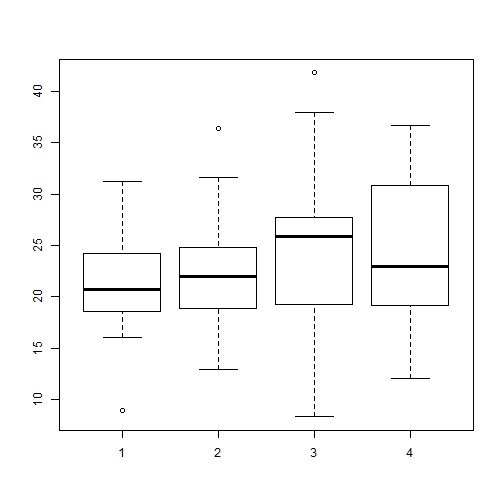Above I generate 100 random normal values, 25 each from four distributions: N(22,5), N(23,5), N(24,8) and N(25,8). Then I generate a 4-level grouping variable. Finally I make the boxplot. The black lines in the “middle” of the boxes are the median values for each group. For group 1, that appears to be a shade above 20. The vertical size of the boxes are the interquartile range, or IQR. They measure the spread of the data, sort of like standard deviation. The tops and bottoms of the boxes are referred to as “hinges”. We see groups 1 and 2 have less variability than groups 3 and 4, which makes sense given the way we generated the data. The IQR for group 1 looks to be about 5 judging from the height of the box. Again this makes sense given group 1 data was randomly generated from a normal distribution with a standard deviation of 5. And now we come to the “whiskers”, or the flattened arrows extending out of the box. What do they represent and how are they calculated? They represent the reasonable extremes of the data. That is, these are the minimum and maximum values that do not exceed a certain distance from the middle 50% of the data. What is that certain distance? By default in R, it's $$1.5 \times IQR$$. If no points exceed that distance, then the whiskers are simply the minimum and maximum values. That's the case in group 4. If there are points beyond that distance, the largest point that does not exceed that distance becomes the whisker. And the points beyond the distance are plotted as single points. For groups 1 through 3, there appear to be single points beyond the whiskers. (I say “appear to be single” because technically we could have overplotting.) We might think of these as outliers, data points that are too big or too small compared to the rest of the data. Group 4 does not appear to have outliers. When you create a boxplot in R, you can actually create an object that contains the plotted data. Just call the boxplot as you normally would and save to a variable. bp <- boxplot(y ~ x, plot = F) bp ##$stats
##          [,1]     [,2]      [,3]     [,4]
## [1,] 16.06564 12.90309  8.300651 12.05522
## [2,] 18.53334 18.90152 19.281150 19.14307
## [3,] 20.75958 21.98459 25.924704 23.00494
## [4,] 24.18153 24.84778 27.721310 30.84133
## [5,] 31.22629 31.65432 38.016463 36.66171
##
## $n ##  25 25 25 25 ## ##$conf
##          [,1]     [,2]     [,3]     [,4]
## [1,] 18.97475 20.10557 23.25761 19.30830
## [2,] 22.54441 23.86361 28.59179 26.70159
##
## $out ##  8.911472 36.409950 41.843672 ## ##$group
##  1 2 3
##
## $names ##  "1" "2" "3" "4" We see the bp object is a list with 6 different elements. The first element, stats, contains the plotted points in each group. So looking at column 1, we see that the bottom and top whiskers are 16.0656374 and 31.226286, the 25th and 75th percentiles are 18.5333406 and 24.1815345, and the median is 20.759577. The out element of the bp object contains the outliers while the group element contains the outliers' respective groups. If you want to change how whiskers are determined, you can change the range argument in the boxplot function. The default setting is 1.5. Here we try it set to 1: boxplot(y ~ x, range=1)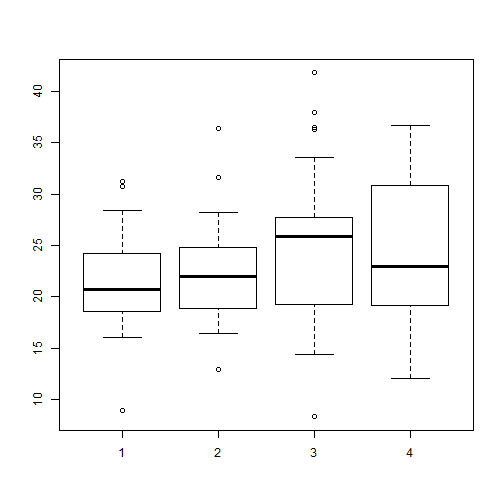Now we have more “outliers” for groups 1 – 3 but still none for group 4. Obviously the “outlier” classification is subjective. You'll also notice the size of the boxes didn't change. They will always capture the middle 50% of the data no matter the value of range. If we set range to 0, then the whiskers will extend to the minimum and maximum values for each group. In that case you get a plot of what is known as Tukey's Five-number summary: minimum, 25th percentile, median, 75th percentile and the maximum. In fact there's a function in R to calculate the Five-Number summary called fivenum. Here's how we can use it on our data: tapply(y,x,fivenum) ##$1
##   8.911472 18.533341 20.759577 24.181534 31.226286
##
## $2 ##  12.90309 18.90152 21.98459 24.84778 36.40995 ## ##$3
##   8.300651 19.281150 25.924704 27.721310 41.843672
##
## $4 ##  12.05522 19.14307 23.00494 30.84133 36.66171 boxplot(y ~ x, range=0)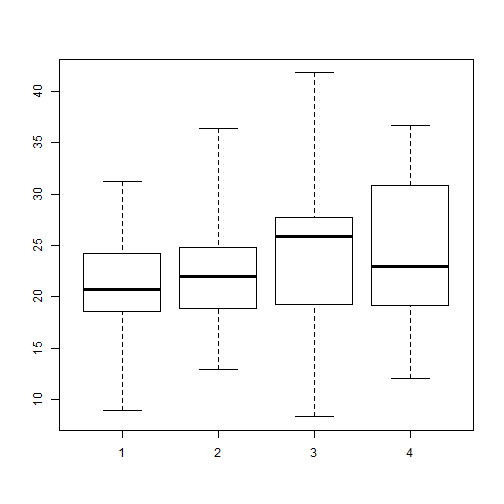A nice complement to boxplots are stripcharts. These are one-dimensional scatter plots of data. Here's out it works “out of the box”: stripchart(y ~ x)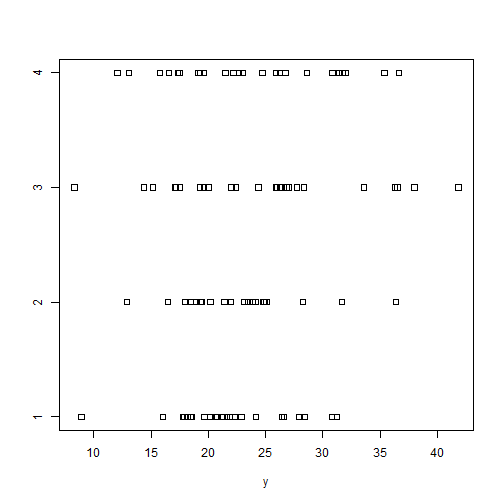I usually like to rotate stripcharts and use the familiar open circles with some “jitter”: stripchart(y ~ x, vertical = TRUE, pch=1, method="jitter")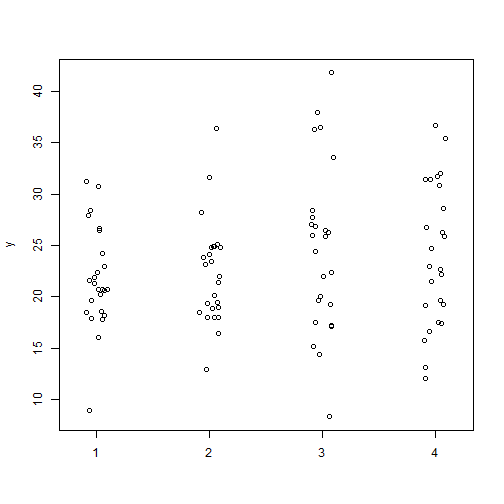Plotting stripcharts and boxplots side-by-side can be useful to visualize the spread and distribution of data. You can also calculate means and medians add them with the points function: op <- par(mfrow=c(1,2)) means <- tapply(y,x,mean) # calculate means medians <- tapply(y,x,median) # calculate medians boxplot(y ~ x, ylab="y") points(x = 1:4,y=means,pch=19) # add means stripchart(y ~ x, vertical = TRUE, pch=1, method="jitter") points(x = 1:4,y=means,pch=19, col="blue") # add means points(x = 1:4,y=medians,pch=19, col="red") # add means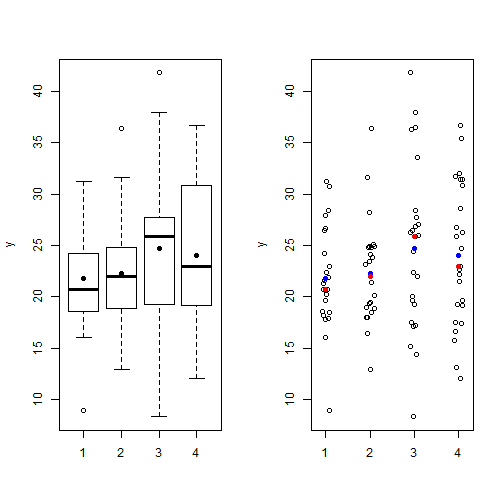par(op) # reset graphics parameters Poisson regression – Ch 6 of Gelman and Hill Chapter 6 of Gelman and Hill's Data Analysis Using Regression and Multilevel/Hierarchical Models presents an interesting example of Poisson regression using data on police stops in New York. Put simply, they seek to model the count of “stop and frisks” as a function of ethnicity and precinct with number of arrests in the previous year included as an offset. While the example is fun and informative, it's not terribly easy to replicate. The R code is provided to run the regressions but the data is a little hard to locate. I was eventually able to find it and get everything to mostly work, so I thought I would blog about it in case anyone else was trying to do the same. About the data: it turns out you can find it here in the “police” folder. In the folder is a dat file called “frisk_with_noise”. Here's one way to read it in: url <- "http://www.stat.columbia.edu/~gelman/arm/examples/police/frisk_with_noise.dat" dat <- read.table(url,header=TRUE, skip=6) head(dat,n=4) ## stops pop past.arrests precinct eth crime ## 1 75 1720 191 1 1 1 ## 2 36 1720 57 1 1 2 ## 3 74 1720 599 1 1 3 ## 4 17 1720 133 1 1 4 dim(dat) ##  900 6 But wait. You'll notice the data set has 900 rows but the regression output in the book references n = 225. To replicate the example in the book, I'm pretty sure we need to aggregate over precinct and eth, like so: stops <- aggregate(cbind(stops, past.arrests) ~ eth + precinct, data=dat, sum) Using cbind() on stops and past.arrests allows us to sum both stops and past.arrests over all combinations of eth and precinct. Now we're ready to run the code provided in the book, which can also be found here. Here's one of the models they fit: library(arm) # for the display() function fit.2 <- glm (stops ~ factor(eth), data=stops, family=poisson, offset=log(past.arrests)) display(fit.2) ## glm(formula = stops ~ factor(eth), family = poisson, data = stops, ## offset = log(past.arrests)) ## coef.est coef.se ## (Intercept) -0.59 0.00 ## factor(eth)2 0.07 0.01 ## factor(eth)3 -0.16 0.01 ## --- ## n = 225, k = 3 ## residual deviance = 45437.4, null deviance = 46120.3 (difference = 682.9) That works, but our output doesn't match the book's output! What's going on? Actually the explanation is in the title of the data file: “frisk_with_noise”. Also notice how I skipped the first 6 lines of the file when reading it in to R. Here's the first line of what I skipped: stop and frisk data (with noise added to protect confidentiality) So noise was apparently added after publication of the book to protect confidentiality. I guess we're not supposed to see which precincts are making the most stops of certain ethnicities? Oh well, at least we can run the code now. We can see fitted values using the fitted() function. Here are the first 10 fitted values compared to their observed values: cbind(observed=stops$stops[1:10], fitted=fitted(fit.2)[1:10])
##    observed    fitted
## 1       202  544.2816
## 2       102  175.7562
## 3        81  180.0316
## 4       132  418.2082
## 5       144  331.8515
## 6        71  203.6578
## 7       752 1215.1920
## 8       441  373.5563
## 9       410  584.9845
## 10      385  261.5884

Doesn't look too good to the eye, at least not the first 10. How are those fitted values calculated? Like this:

$y = exp(\alpha + \beta x + log(t))$

where t is the offset, in this case, past arrrests. So to calculate the fitted value for precinct 1, ethnicity = 1 (black), and 980 arrests in the previous year, we do the following:

exp(coef(fit.2) + log(980))
## (Intercept)
##    544.2816
# or equivalently
980*exp(coef(fit.2))
## (Intercept)
##    544.2816
# compare to R's fitted value
fitted(fit.2) == exp(coef(fit.2) + log(980))
##    1
## TRUE

Plotting standardized residuals versus fitted values can reveal overdispersion. That's what Gelman and Hill do in figure 6.1. Below I reproduce it. First I fit a new model that includes precinct as a predictor. The figure on the left plots raw residuals versus fitted values. This is of limited use since we expect variance to increase with larger fitted values in a Poisson distribution. In other words we wouldn't expect to see a constant scatter about 0 as we would in OLS regression. The figure on the right, however, uses standardized residuals which have a mean of 0 and standard deviation of 1. If the Poisson model is true, we would expect to see most residuals falling within 2 standard deviations from 0. Many residuals falling beyond this range reveal overdispersion. And indeed that's what we see here.

fit.3 <- glm (stops ~ factor(eth) + factor(precinct), data=stops, family=poisson, offset=log(past.arrests))
par(mfrow=c(1,2))
pv <- fitted(fit.3)
r <- (stops$stops - fitted(fit.3)) plot(pv, r, pch=20, ylab="raw residuals", xlab="predicted value") abline(h=0) sr <- (stops$stops - fitted(fit.3))/sqrt(fitted(fit.3))
plot(pv, sr, pch=20, ylab="standardized residuals", xlab="predicted value")
abline(h=c(-2,0,2),lty=c(2,1,2))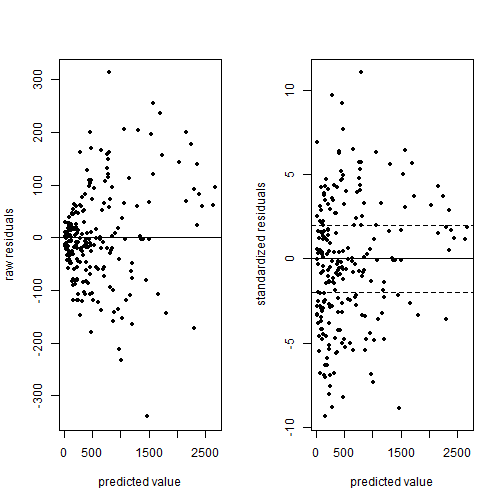par(mfrow=c(1,1))

To correct for overdisperson in R you can repeat the code for fit.3 above with family = quasipoisson. This corrects the standard errors of the regression coefficients by making them larger.

Understanding Mosaic Plots

Mosaic plots provide a way to visualize contingency tables. However they’re not as intuitive as, say, a scatter plot. It’s hard to mentally map a contingency table of raw counts to what you’re seeing in a mosiac plot. Let’s demonstrate.

Here I use data from Example 8.6-3 of Probability and Statistical Inference (Hogg & Tanis, 2006). These data are a random sample of 400 University of Iowa undergraduate students. The students were classified according to gender and the college in which they were enrolled.

M <- matrix(data = c(21,14,16,4,145,175,2,13,6,4),
ncol=5,
dimnames = list(Gender=c("Male","Female"),

M
##         College
## Gender   Business Engineering Liberal Arts Nursing Pharmacy
##   Male         21          16          145       2        6
##   Female       14           4          175      13        4

To create a basic mosaic plot in R, you use the mosaicplot function with a contingency table as the first argument. This can be a table or matrix object. I’ve also added a title to the graph and used the las argument to make the axis labels horizontal.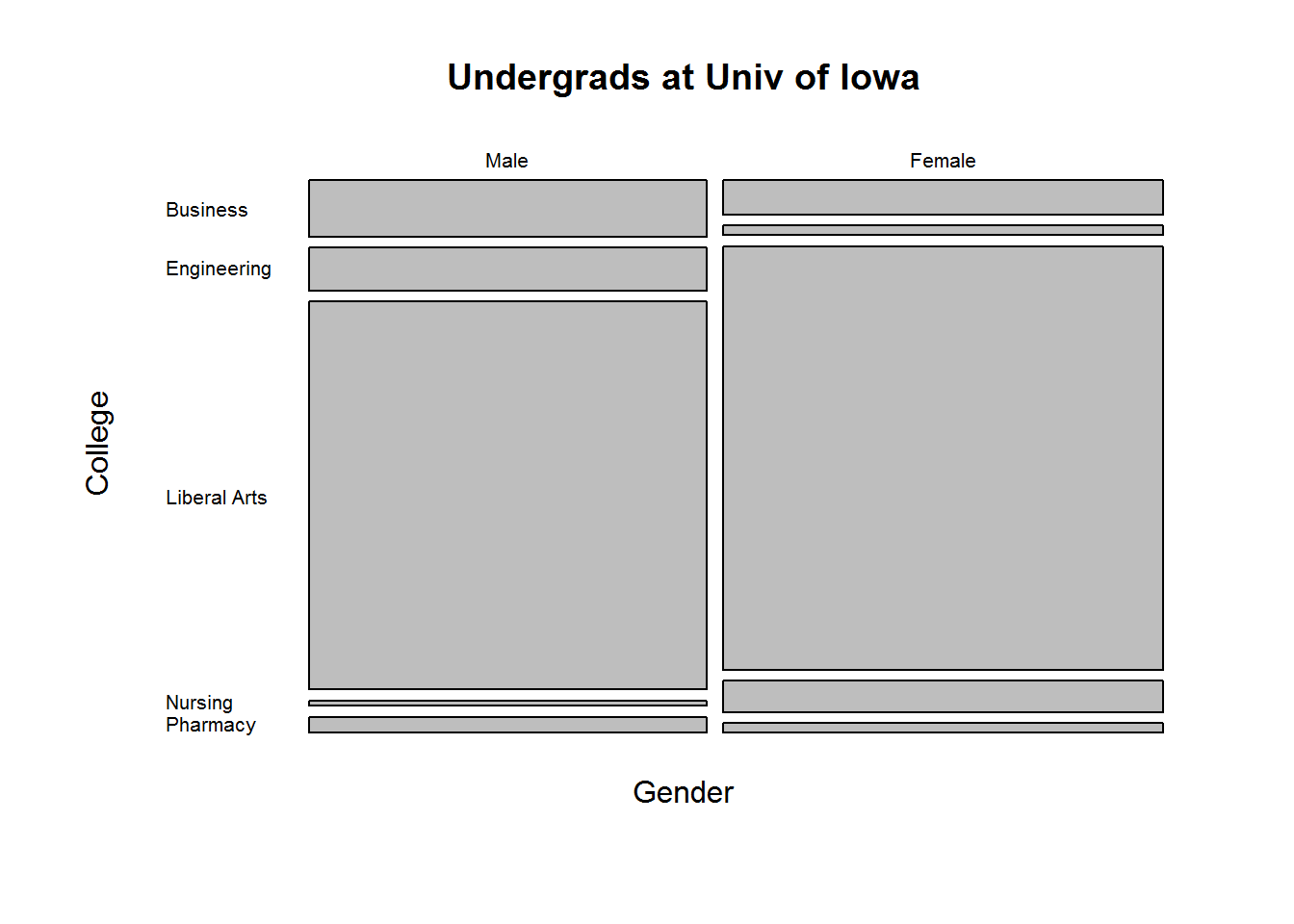The main feature that likely jumps out to you is the lack of numbers. We just see rectangles stacked on one another. If we compare the mosaic plot to the table of counts, the size of the boxes seem related to the counts in the table. Indeed they are, but how? Another feature you may notice is that the widths of the rectangles do not vary but the heights do. What’s up with that?

By default, the mosaicplot function recursively calculates marginal proportions starting with the rows. In our example, we start with gender:

apply(M, 1, function(x)sum(x)/sum(M))
##   Male Female
##  0.475  0.525

That simply says 0.475 of our sample is male, and 0.525 is female. These are the widths of the rectangles.

Now within gender, calculate the proportion belonging to each college:

prop.table(M, margin = 1)
##         College
## Gender     Business Engineering Liberal Arts    Nursing   Pharmacy
##   Male   0.11052632  0.08421053    0.7631579 0.01052632 0.03157895
##   Female 0.06666667  0.01904762    0.8333333 0.06190476 0.01904762

Among males about 11% are enrolled in the Business college versus only 6% among females. These are the heights of our rectangles.

The scale of the plot is basically 0 to 1 on the x and y axes. So the first rectangle we see in the Male column for the Business college is 0.475 wide and about 0.11 tall. In the Female column, the rectangle for the Business college is 0.525 wide and about 0.07 tall. Visually we see there are more Females than Males in our sample because the Female rectangles are wider. Within the gender columns, we see Males have a higher proportion in the Business school than do Females because their rectangle is taller.

That’s what mosaic plots attempt to visualize: recursive proportions of membership within a n-dimension table.

Let’s try it on a table with 3 dimensions. Below we’ll use a data set that comes with R called UCBAdmissions. This data set contains “aggregate data on applicants to graduate school at Berkeley for the six largest departments in 1973 classified by admission and sex.” This is a rather famous data set used for illustrating Simpson’s Paradox.

## , , Dept = A
##
##           Gender
##   Rejected  313     19
##
## , , Dept = B
##
##           Gender
##   Rejected  207      8
##
## , , Dept = C
##
##           Gender
##   Rejected  205    391
##
## , , Dept = D
##
##           Gender
##   Rejected  279    244
##
## , , Dept = E
##
##           Gender
##   Rejected  138    299
##
## , , Dept = F
##
##           Gender
##   Rejected  351    317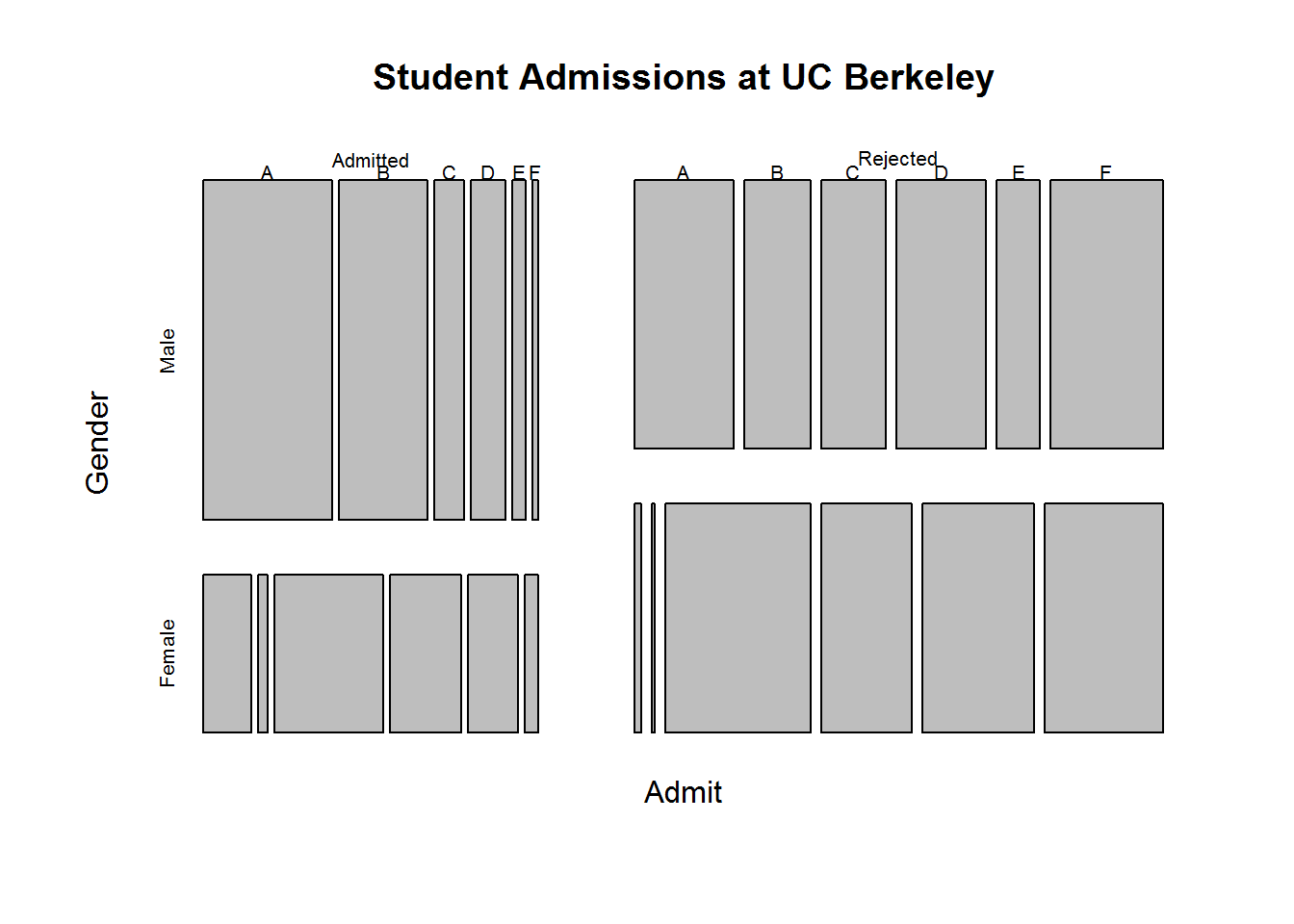Were Depts A and B discriminating against Females? You might think so if you stop there. But look at the Rejected column. We see that of the rejected Males and Females, a much higher proportion of the Males were rejected for Depts A and B than Females. The widths of the Male rectangles are wider than their Female counterparts. Likewise for Depts C – F. It’s pretty clear that of the rejected Males and Females, a higher proportion of the Females were rejected for Depts C – F than Males. Again the widths of the Female rectangles are wider than their Male counterparts.

That’s where Simpson’s Paradox comes into play. If we disregard the within Dept counts, we see what appears to be Female discimination:

# collapse count over departments and create mosaic plot
##           Gender
##   Rejected 1493   1278
main = "Student admissions at UC Berkeley")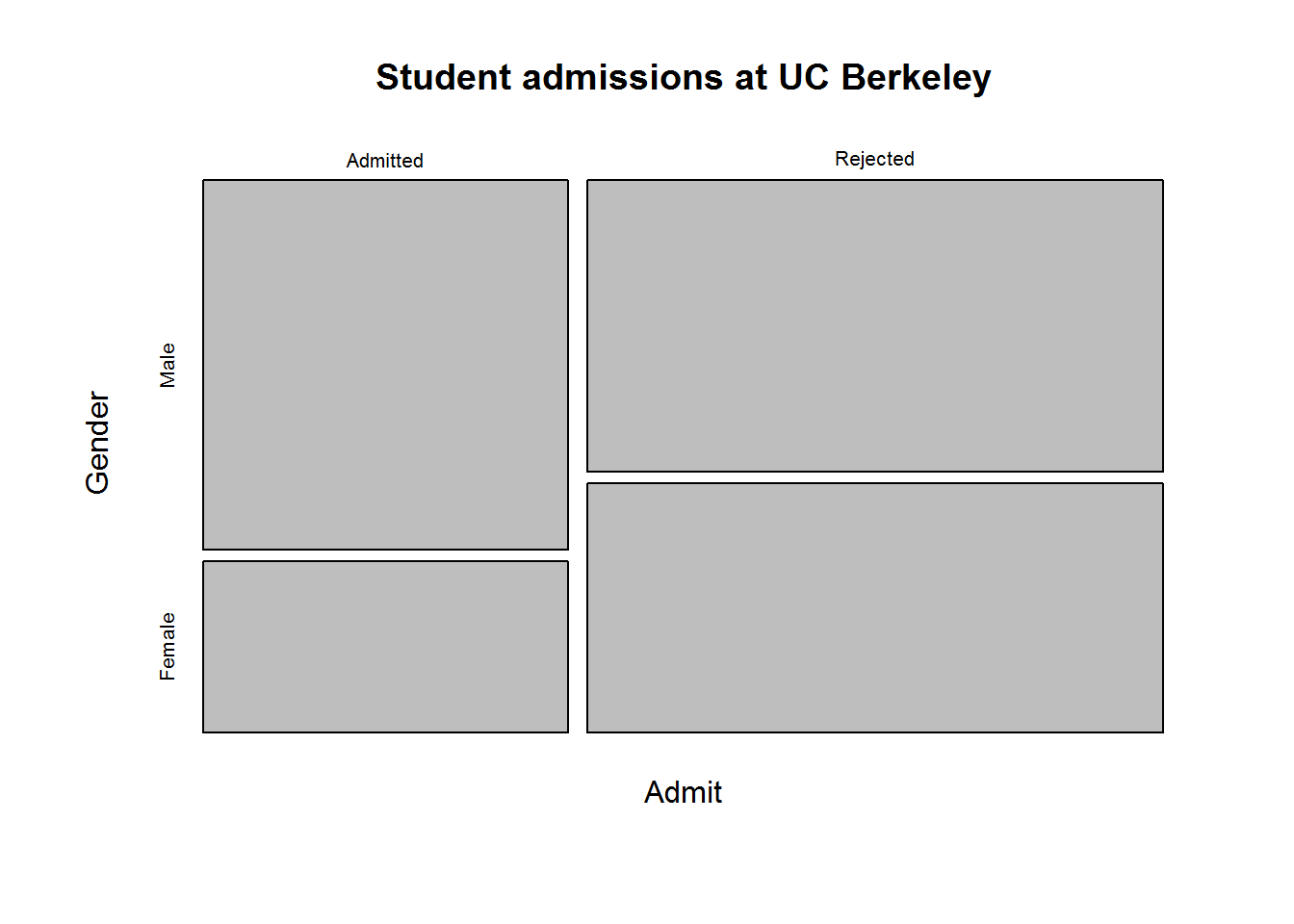To really understand what mosaic plots are showing, it helps to create one “by hand”. There’s no real point in doing so other than personal edification. But let’s be edified. We’ll work with our Univ of Iowa data.

We know our plot needs x and y axes with a scale of 0 to 1. We also know we need to draw rectangles. Fortunately R has a rect function that allows you to create rectangles. You tell it the coordinate points for the bottom left and upper right corners of your rectangle and it does the rest.

In order to translate the width and height of rectangles to locations within the plot, we’ll need to use the cumsum function. I need to draw rectangles relative to other rectangles. Hence the position of a rectangle corner will need to take into account other rectangles drawn above or beside it. The cumsum function allows us to do that.

Here’s my rough stab at a manual mosaic plot:

# widths
widths <- cumsum(c(0, apply(M, 1, function(x)sum(x)/sum(M))))
# heights
pt <- prop.table(M,margin = 1)
heightsM <- cumsum(c(0,pt[1,]))
heightsF <- cumsum(c(0,pt[2,]))
# Need to reverse the y axis
plot(x=c(0,1), y=c(0,1), xlim=c(0,1), ylim=c(1,0),
type = "n", xlab = "", ylab = "")
# male rectangles
rect(xleft = widths, ybottom = heightsM[-1],
xright = widths, ytop = heightsM[-6], col=gray(seq(3,12,2) / 15))
# female rectangles
rect(xleft = widths, ybottom = heightsF[-1],
xright = widths, ytop = heightsF[-6], col=gray(seq(3,12,2) / 15))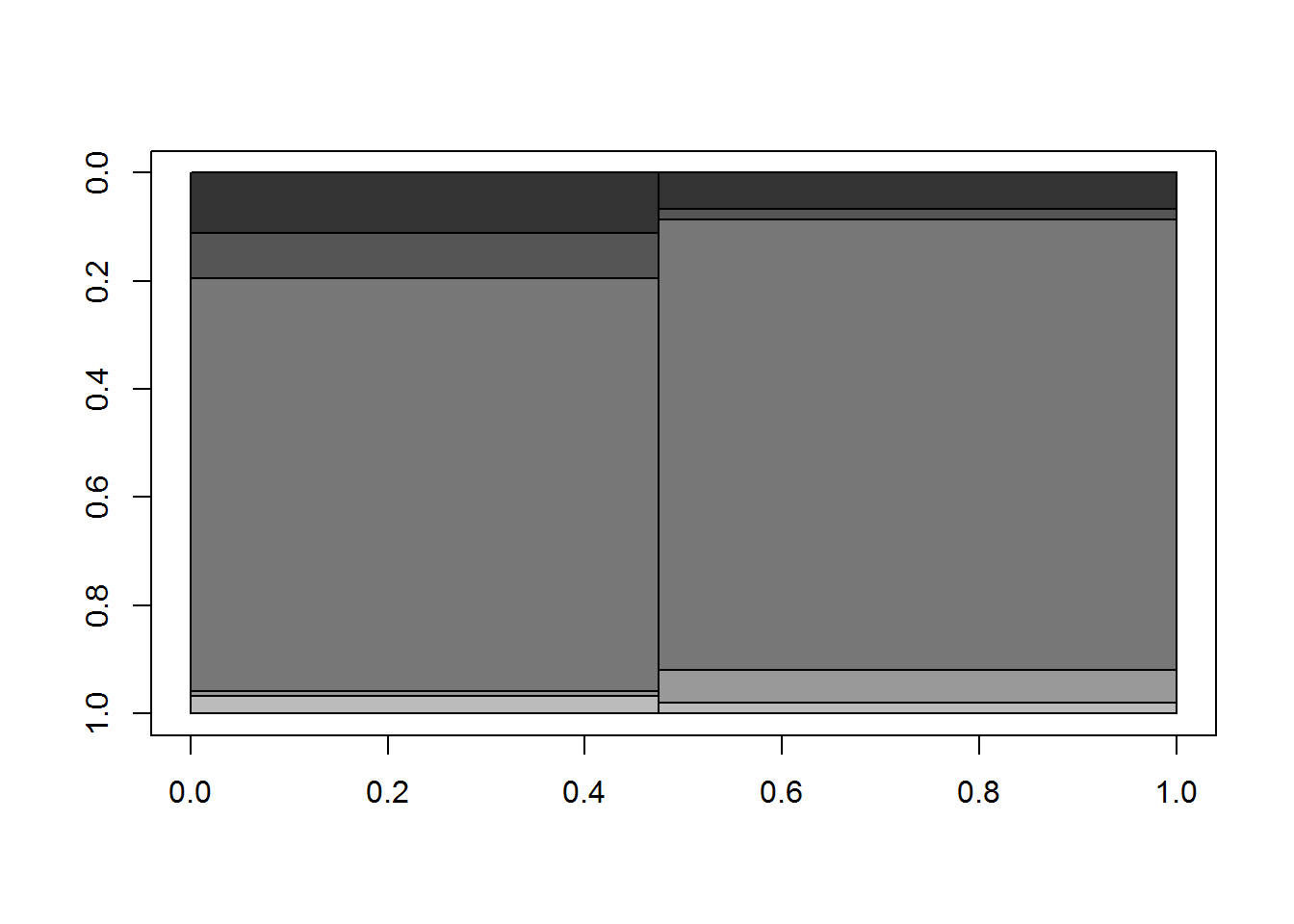If you compare that to the original mosaicplot() output above that I drew at the beginning of this post you can see we’ve basically drawn the same thing without spacing around the rectangles. That’s why I used the gray function to fill in the boxes with distinguishing shades. Again, nowhere near as nice as what the mosaicplot give us, but a good way to understand what the mosaicplot function is doing.

Review of R Graphs Cookbook

I was recently invited to review the second edition of the R Graphs Cookbook by Jaynal Abedin and Hrishi V. Mittal (Packt Publishing). This is not to be confused with the R Graphics Cookbook by Winston Chang (O’Reilly Media). I own the latter and refer to it on a regular basis. I was curious to see how the R Graphs Cookbook would compare to it.

The book has 15 chapters. The first 8 cover how to do traditional graphs such as scatter plots, line graphs, histograms, box plots and the like along with extensive coverage of tweaking graphical parameters. Chapters 9 – 14 cover special topics like heat maps, geographical maps, lattice and ggplot2. The final chapter presents several recipes for finalizing graphs for publication.

Each “recipe” follows a template of first showing How to Do It followed by a How it Works section that walks you through the code. There’s also a There’s More section that offers extra tips or suggestions and finally a See Also section that refers you to similar recipes. The recipes frequently use sample data sets that need to be downloaded from the Packt Publishing site. Otherwise they tend to use data sets included with the base R datasets package or randomly generated data. Each recipe includes the graph that it produces in color, at least they do in the the PDF version I reviewed. (I don’t know if the paperback includes color. For the asking price of $46 on Amazon I hope it does.) My overall impression of the book is positive. The recipes are practical, the explanations of code are clear, and there are several pointers to useful R packages.The chapters that really stood out to me (ie, chapters I could see myself using) were the coverage of graphical parameters in chapter 3, working with map and GIS data in chapter 10, and preparing graphs for publication in chapter 15. But the book isn’t perfect. My biggest complaint is the book datasets. They’re in a folder structure that doesn’t always match the book’s contents. For example there is a health expenditure dataset in the Chapter 3 folder that is not used until Chapter 4. As another example, the recipe for choosing plot symbols and sizes asks us to load the “cityrain.csv” data “that we used in the first chapter.” But it’s not used in the first chapter, it’s used it in the second chapter. But in this case the dataset is actually in the Chapter 2 folder! I frequently found myself setting my working directory to use a chapter’s dataset only to find the dataset wasn’t there. All this could have been avoided by just lumping all data into a single folder. Or perhaps by making an R package that contains the book’s datasets as the author of the R Graphics Cookbook has done. Another head-scratcher is the introduction to the grid package in the first section of Chapter 1. The section is titled “Base graphics using the default package”, yet grid is presented as the base graphics package. It’s not. The base R graphics package is the graphics package. The authors clearly know a great deal about creating R graphics, but I don’t understand their reasoning for presenting the grid package as the default package for graphs. There are a few recipes that I think could be improved. The “Formatting log axes” recipe simply plots$10^1$ through$10^5$ with the log argument set to “y”. Why not use one of the book’s datasets and show a before and after graph to demonstrate how the axis changes with log formatting? For example: > metals <- read.csv("Chap 3/Data Files/metals.csv") > par(mfrow=c(1,2)) > plot(Ba~Cu,data=metals,xlim=c(0,100), main="Before y-axis\n log transformation") > plot(Ba~Cu,data=metals,xlim=c(0,100), log="y", main="After y-axis\nlog transformation") > par(mfrow=c(1,1))The “Creating bar charts with vertical error bars” recipe in chapter 6 creates error bars by multiplying the plotted values by 0.95 and 1.05. Why not show how to plot actual standard error bars? In fact they conclude the recipe by saying “In practice, scaled estimated standard deviation values or other formal estimates of error would be used to draw error bars instead of a blanket percentage error as shown here.” To their credit, the authors do show how to create conventional standard error bars later on in the ggplot2 chapter. At the very least it seems like the recipe in chapter 6 should have a See Also section that points readers to the ggplot2 recipe. One other recipe I thought could be better was “Setting graph margins and dimensions”. It tells you how to do it but doesn’t actually demonstrate it. It would have been nice to see the effect of changing the various parameters. In fact I’m still not sure how the fin and pin par() arguments work. Of course the authors go on to say “it is better to use mar or mai” instead of fin and pin, which I suppose is nice since I know how mar and mai work. But then why mention fin and pin in the first place? While I’m on the subject of setting graphics parameters I noticed the authors never explicitly explain how to restore initial par() values by saving the result of par() when making changes. For example, > oldpar <- par(col=4, lty=2) … plotting commands … > par(oldpar) They do it once in chapter 1 when demonstrating trellis graphs but they don’t explain what it’s doing or why it’s there. I really believe that should be a separate recipe in chapter 3, “Beyond the Basics – Adjusting Key Parameters”. Some of the recipes I really liked were “Setting fonts for annotations and titles”, “Using margin labels instead of legends for multiple-line graphs” and “Showing the number of observations” in the axis labels of box plots. Those three are golden. The recipe for “Graph annotation with ggplot” is also quite useful. And I thoroughly enjoyed working through the “Data Visualization Using Lattice” chapter. I had never used Lattice before and found this to be an excellent tutorial. As I mentioned earlier, I own and make regular use of O’Reilly’s R Graphics Cookbook. How does Packt’s R Graphs Cookbook compare? The main difference is ggplot2. The O’Reilly book is almost exclusively devoted to ggplot2. In fact if not for the Miscellaneous Graphs chapter near the end it could very easily be called the ggplot2 Cookbook. The R Graphs Cookbook on the other hand is rooted in base R graphics. ggplot2 gets introduced briefly in chapters 1 and 4 before getting its own treatment in chapter 12. If you’re looking for a ggplot2 reference, O’Reilly’s R Graphics Cookbook is hands-down the best choice. But if you want a reference for base R graphics, then the R Graphs Cookbook is the better of the two. Setting up a keyboard shortcut for the dplyr chain operator I finally reached the point where I was using dplyr enough to get annoyed with typing %>%. I’m guessing if I was using Linux and Emacs this would be a trivial problem to solve. But I use RStudio on Windows, so my solution is a little more involved. Here it is in case anyone is interested. 1. Go to http://www.autohotkey.com/, download AutoHotkey, and install. It’s open source. 2. Right click on your desktop and click New > AutoHotkey Script 3. Give it name like “chain” 4. Right click on the script you just created and click Edit Script 5. Leave the existing text in the script as is and enter the following at the bottom, which maps %>% to the keys Ctrl + Shift + . (period) ^+.:: SendRaw % SendRaw > SendRaw % return 6. Save and close the file 7. Double-click on the file; it should now be running in your system tray 8. Go to RStudio and hit Ctrl + Shift + . (period) That should enter %>% To make this happen every time you start your computer, move or copy the “chain.ahk” file to your Startup folder. To learn more about all the things AutoHotkey can do, check out their documentation. Credit where credit is due: Here is the Stack Overflow thread where I learned about Autokey, which led me to AutoHotkey. And here is a helpful exchange on the AutoHotkey forum that showed me how to get the script to work. UPDATE: LOL, turns out there’s a hot-key in R studio for this: Ctrl + Shift + M. Partial Residuals and the termplot function Earlier this year I taught an Intro to R Graphics workshop. While preparing for it I came across the termplot function. I had no idea it existed. Of course now that I know about it, I come across it fairly regularly. (That's the Baader-Meinhof Phenomenon for you.) ?termplot will tell you what it does: “Plots regression terms against their predictors, optionally with standard errors and partial residuals added.” If you read on, you'll see “Nothing sensible happens for interaction terms, and they may cause errors.” Duly noted. Let's try this out and see what it does. First we'll fit a model using the mtcars dataset that comes with R. Below mpg = Miles/(US) gallon, drat = Rear axle ratio, qsec = ¼ mile time, disp = Displacement (cu.in.), and wt = Weight (lb/1000). lm.cars <- lm(mpg ~ wt + disp + drat + qsec, data=mtcars) summary(lm.cars) ## ## Call: ## lm(formula = mpg ~ wt + disp + drat + qsec, data = mtcars) ## ## Residuals: ## Min 1Q Median 3Q Max ## -4.143 -1.933 -0.149 0.919 5.541 ## ## Coefficients: ## Estimate Std. Error t value Pr(>|t|) ## (Intercept) 9.03223 9.58829 0.94 0.35454 ## wt -4.86768 1.20872 -4.03 0.00041 *** ## disp 0.00522 0.01105 0.47 0.64021 ## drat 1.87086 1.32462 1.41 0.16926 ## qsec 1.05248 0.34778 3.03 0.00539 ** ## --- ## Signif. codes: 0 '***' 0.001 '**' 0.01 '*' 0.05 '.' 0.1 ' ' 1 ## ## Residual standard error: 2.6 on 27 degrees of freedom ## Multiple R-squared: 0.838, Adjusted R-squared: 0.814 ## F-statistic: 35 on 4 and 27 DF, p-value: 2.55e-10 par(mfrow=c(2,2)) termplot(lm.cars)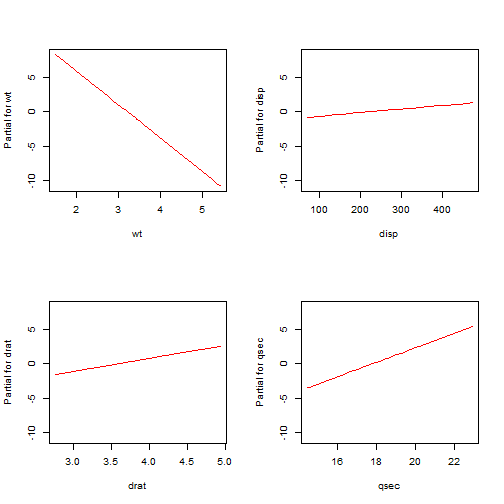Compare the coefficients in the summary output to the slopes of the lines in the graphs. See how the coefficients match the slopes of the lines? That's because they are the slopes of the lines. For example, wt has a coefficient of about -5. Likewise the termplot for that coefficient has a negative slope that appears to be about -5. Using these plots we can see at a glance the respective contributions of the predictors. wt and qsec seem to contribute to the model due to the steepness of their slopes, but disp and drat not so much. This matches the summary output that shows wt and qsec as being significant. Now termplot also has an argument called partial.resid that will add partial residuals if set to TRUE. Let's try that out, with color set to “purple” since the default gray can be hard to see (at least to my eyes): par(mfrow=c(2,2)) termplot(lm.cars, partial.resid=TRUE, col.res = "purple")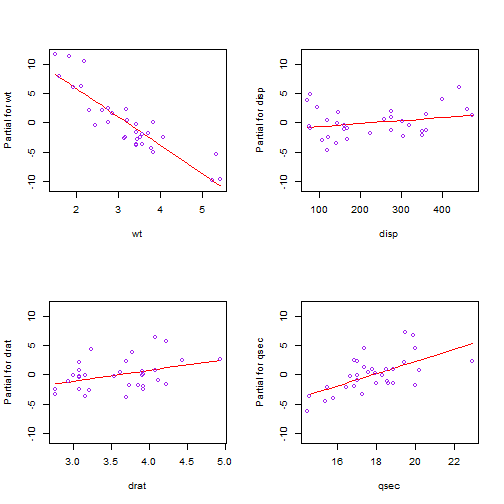How are these points generated? The x-axis is clear enough. That's just the predictor values. But the y-axis says “Partial for [variable name]”, i.e. partial residuals. What does that mean? Put as simply as possible, partial residuals are the sum of the residuals and predictor terms. Hopefully you know what residuals are. Those are just the difference between the observed and fitted response values. “Predictor terms” is a little trickier. To understand them, it helps to know that $y = \bar{y} + b_{1}(x_{1} – \bar{x}_{1}) + b_{2}(x_{2} – \bar{x}_{2}) + b_{3}(x_{3} – \bar{x}_{3}) + b_{4}(x_{4} – \bar{x}_{4}) + e$ is another way of writing $y = b_{0} + b_{1}x_{1} + b_{2}x_{2}+ b_{3}x_{3}+ b_{4}x_{4} + e$ So the first equation above has $$\bar{y}$$ as the intercept and centered predictors. If we center the predictors and fit a model, we'll see that's precisely what we get: # center the variables mtcars <- transform(mtcars, wtC = wt-mean(wt), dispC = disp-mean(disp), dratC=drat-mean(drat), qsecC=qsec-mean(qsec)) # now fit model using centered predictors lm.terms.cars <- lm(mpg ~ wtC + dispC + dratC + qsecC, data=mtcars) coef(lm.terms.cars) ## (Intercept) ## 20.09 mean(mtcars$mpg)
##  20.09

Now if we take the centered predictor values and multiply them by their respective coefficients, we get what are referred to in R as terms. For example, the terms for the first record in the data set are as follows:

# take all coefficients but the intercept...
coef(lm.terms.cars)[-1]
##       wtC     dispC     dratC     qsecC
## -4.867682  0.005222  1.870855  1.052478
# ...and first record of data...
lm.terms.cars$model[1,-1] ## wtC dispC dratC qsecC ## Mazda RX4 -0.5972 -70.72 0.3034 -1.389 # ...and multiply: coef(lm.terms.cars)[-1]*t(lm.terms.cars$model[1,-1])
##       Mazda RX4
## wtC      2.9072
## dispC   -0.3693
## dratC    0.5677
## qsecC   -1.4616

So for the first record, the term due to wt is 2.91, the term due to disp is -0.37, and so on. These are the predictor terms I mentioned earlier. These are what we add to the residuals to make partial residuals.

You'll be happy to know we don't have to center predictors and fit a new model to extract terms. R can do that for us on the original model object when you specify type="terms" with the predict function, like so:

# notice we're calling this on the original model object, lm.cars
pterms <- predict(lm.cars, type="terms")
pterms[1,] # compare to above; the same
##      wt    disp    drat    qsec
##  2.9072 -0.3693  0.5677 -1.4616

To calculate the partial residuals ourselves and make our own partial residual plots, we can do this:

# add residuals to each column of pterms (i.e., the terms for each predictor)
partial.residuals <- apply(pterms,2,function(x)x+resid(lm.cars))
# create our own termplot of partial residuals
par(mfrow=c(2,2))
# the model in lm.cars includes the response in first column, so we index with i + 1
for(i in 1:4){
plot(x=lm.cars$model[,(i+1)],y=partial.residuals[,i], col="purple", ylim=c(-10,10)) abline(lm(partial.residuals[,i] ~ lm.cars$model[,(i+1)]), col="red")
}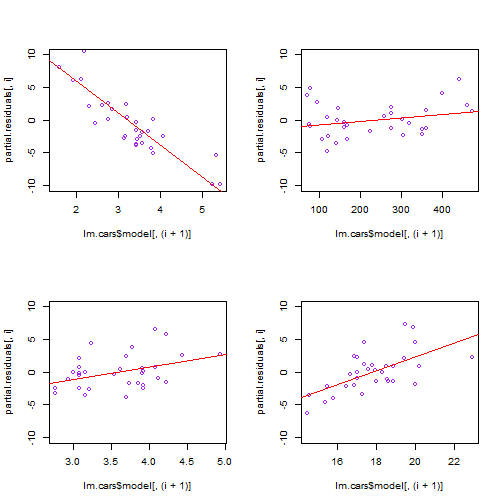So that's what termplot does for us: it takes the terms for each predictor, adds the residuals to the terms to create partial residuals, and then plots partial residuals versus their respective predictor, (if you specify partial.resid=TRUE). And of course it plots a fitted line, the result of regressing the predictor's partial residuals on itself. Recall ealier I mentioned the slope of the line in the termplot graphs is the coefficient in the summary output. We can indeed verify that:

# coefficient for wt
coef(lm.cars)
##     wt
## -4.868
coef(lm(partial.residuals[,1] ~ mtcars$wt)) ## mtcars$wt
##    -4.868

Ultimately what this whole partial residual/termplot thing is doing is splitting the response value into different parts: an overall mean, a term that is due to wt, a term that is due to disp, a term that is due to drat, and a term that is due to qsec. And you have the residual. So when we create the partial residual for wt, what we're doing is adding the wt term and the residual. This sum accounts for the part of the response not explained by the other terms.

Again let's look at the overall mean, the terms for the first observation and its residual:

# overall mean of response
mean(mtcars$mpg) ##  20.09 # terms of first observation pterms[1,] ## wt disp drat qsec ## 2.9072 -0.3693 0.5677 -1.4616 # the residual of the first observation resid(lm.cars) ## Mazda RX4 ## -0.7346 If we add those up, we get the observed value # add overall mean, terms and residual for first observation mean(mtcars$mpg) + sum(pterms[1,]) + resid(lm.cars)
## Mazda RX4
##        21
# same as observed in data
mtcars$mpg ##  21 So the wt term value of 2.9072 represents the part of the response that is not explained by the other terms. Finally, we can add another argument to termplot, smooth=panel.smooth that will draw a smooth lowess line through the points. This can help us assess departures from linearity. par(mfrow=c(2,2)) termplot(lm.cars, partial.resid=TRUE, col.res = "purple", smooth=panel.smooth)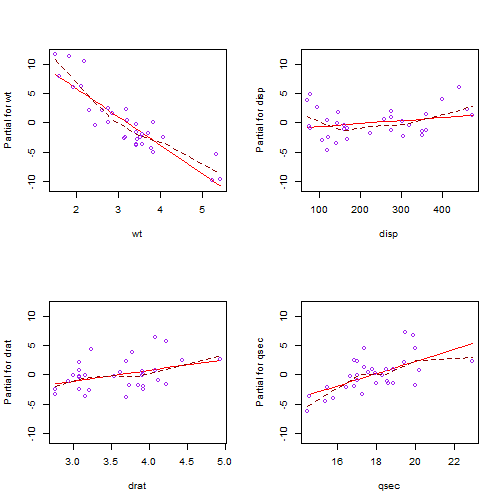Notice how the dashed line bends around the straight line for wt. Perhaps wt requires a quadratic term? Let's try that using the poly function, which creates orthogonal polynomials. lm.cars2 <- lm(mpg ~ poly(wt,2) + disp + drat + qsec, data=mtcars) par(mfrow=c(2,2)) termplot(lm.cars2, partial.resid=TRUE, col.res = "purple", smooth=panel.smooth)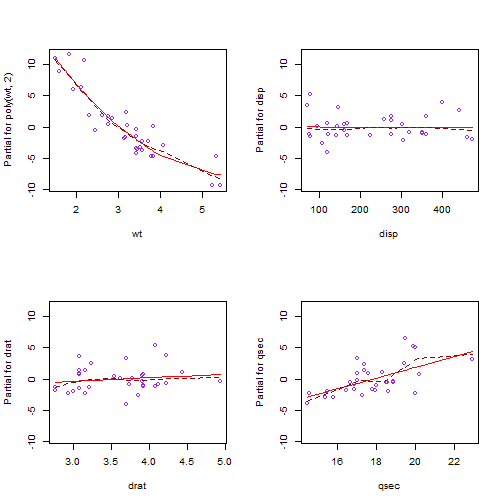We now see a better fit with the regressed line matching closely to the smooth line. Permutation Tests Let's talk about permutation tests and why we might want to do them. First think about the two-sample t-test. The null hypothesis of this test is that both samples come from the same distribution. If we assume both samples come from the same approximately normal distribution, we can use math formulas based on probability theory to calculate a test statistic. We can then calculate the probability of getting such a test statistic (or one greater) under the assumption of both samples coming from the same distribution. For example, let's draw two samples from the same normal distribution and run a t-test. set.seed(135) s1 <- round(rnorm(10, 100, 16)) s2 <- round(rnorm(10, 100, 16)) t.test(s1, s2, var.equal = TRUE) ## ## Two Sample t-test ## ## data: s1 and s2 ## t = 0.8193, df = 18, p-value = 0.4233 ## alternative hypothesis: true difference in means is not equal to 0 ## 95 percent confidence interval: ## -7.508 17.108 ## sample estimates: ## mean of x mean of y ## 102.4 97.6 As expected the p-value is high, telling us the difference in their means is not significant. Another interpretation is that the resulting t-test statistic (0.8193) would be likely if both samples came from the same distribution. In real life we don't know with certainty the distribution of the population from which our samples are drawn. We also don't know the variance of the distribution or whether the distribution is even symmetric. We also may be stuck with a small sample size. Fortunately the t-test is pretty robust and usually reliable even when its assumptions are wrong. However, if you have your doubts, you can try a permutation test. In the case our two-sample example above, the permutation test takes all possible combinations of group membership and creates a permutation distribution. In other words, if we assume both samples came from the same population, a data point in group 1 is just as likely to appear in group 2. If we determine all possible permutations, we can calculate our statistic of interest for each permutation and create a distribution. We can then assess where our original statistic falls in this distribution. If it's in the tails then we have evidence that our two samples come from two different populations. Now if your sample sizes are bigger than say, 15, creating the permutations can get computationally expensive very fast. So this is probably not something you want to do unless you're dealing with small samples. Here's how we can do a permutation test “by hand” in R with our fake data: d0 <- mean(s1) - mean(s2) alls <- c(s1, s2) # combine into one vector N <- length(alls) n <- length(s1) p <- combn(N, n) # generate all combinations of n chosen from N dmeans <- numeric(dim(p)) # vector to store means for (i in 1:dim(p)) { dmeans[i] <- mean(alls[p[, i]]) - mean(alls[-p[, i]]) } # plot histogram of all possible difference in means with lines indicating # our original difference hist(dmeans) abline(v = d0, lty = 2) abline(v = -d0, lty = 2)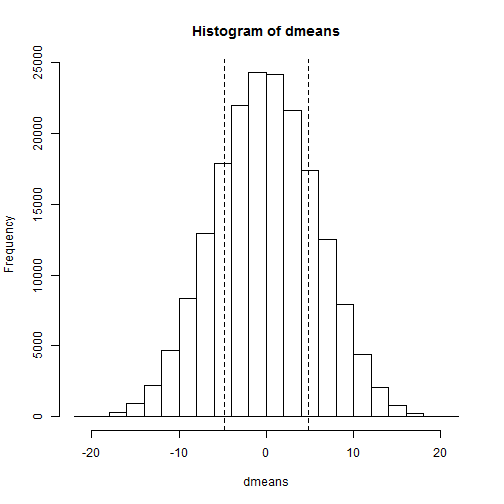The combn function generates a matrix of all possible index combinations of n chosen from N. I say “index” because combn doesn't return the values themselves but rather their index position in the original vector. Thus we can use it to select all possible combinations from our combined vector, which is what we do in the for loop. In this case there are 184756 possible combinations. Once all possible differences in means are calculated we can plot a histogram of the differences and draw some lines to indicate where our original sample falls. Here I drew two lines to indicate the absolute value of the difference, the equivalent of a two-sided test. The probability (or p-value) of getting a difference more extreme than our original sample is pretty high. We can see this because there's a lot of histogram on either side of our lines. We can also calculate this value: # two-sided p-value signif((sum(dmeans <= -abs(d0)) + sum(dmeans >= abs(d0)))/dim(p)) ##  0.4291 Very close to the p-value returned by the t-test. Of course there are packages for this sort of thing. The one I know of is perm. Here's how we use its permTS function to replicate our results above: library(perm) permTS(s1, s2, alternative = "two.sided", method = "exact.ce", control = permControl(tsmethod = "abs")) ## ## Exact Permutation Test (complete enumeration) ## ## data: s1 and s2 ## p-value = 0.4291 ## alternative hypothesis: true mean s1 - mean s2 is 0 ## sample estimates: ## mean s1 - mean s2 ## 4.8 The method= argument tells the function to do “complete enumeration”. The control= argument says how to calculate the p-value (i.e., the same way we did it “by hand”). So that's a literal two-sample permutation test. As I mentioned, if your samples are large then this approach is not feasible as the number of permutations grows out of control. For example, two groups of size 20 results in 137,846,528,820 combinations. So we usually resort to resampling methods. This is where we repeatedly resample from our combined vector of values to get a large number of combinations. We don't generate all combinations, but enough to give us a good idea. Here's one way to do it: dmeans <- numeric(2000) # vector to store means for (i in 1:2000) { g <- sample(N, n) dmeans[i] <- mean(alls[g]) - mean(alls[-g]) } hist(dmeans) abline(v = d0, lty = 2) abline(v = -d0, lty = 2)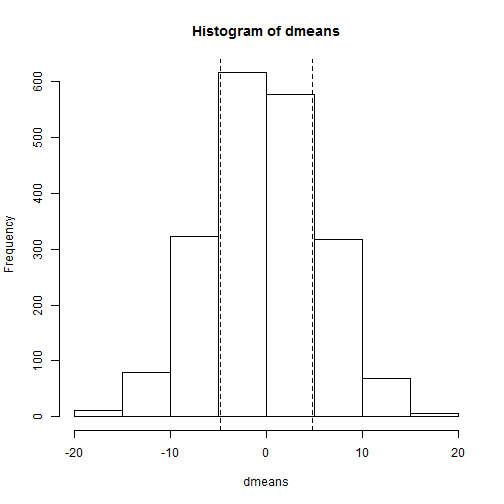signif((sum(dmeans <= -abs(d0)) + sum(dmeans >= abs(d0)))/2000) ##  0.4335 So out of 1.8476 × 105 possible permutations we only calculated 2000 (assuming no repeats) and we still got a p-value pretty close to that of our literal permutation test. Not bad. We can do the same using the permTS function as follows: permTS(s1, s2, alternative = "two.sided", method = "exact.mc", control = permControl(nmc = 2000, setSEED = FALSE, tsmethod = "abs")) ## ## Exact Permutation Test Estimated by Monte Carlo ## ## data: s1 and s2 ## p-value = 0.4213 ## alternative hypothesis: true mean s1 - mean s2 is 0 ## sample estimates: ## mean s1 - mean s2 ## 4.8 ## ## p-value estimated from 2000 Monte Carlo replications ## 99 percent confidence interval on p-value: ## 0.3925 0.4498 The method “exact.mc” says use Monte Carlo simulation. The nmc= argument specifies number of replications (the default is 999). The setSEED= argument says not to set a seed. I want different random replications each time I run this line of code. To wrap up, when does it make sense to use permutation tests? When you have something to permute! I quote from the classic text, An Introduction to the Bootstrap: Permutation methods tend to apply to only a narrow range of problems. However when they apply, as in testing F = G in a two-sample problem, they give gratifyingly exact answers without parametric assumptions. Simulating responses from a linear model Say you fit a model using R's lm() function. What you get in return are coefficients that represent an estimate of the linear function that gave rise to the data. The assumption is the response of the model is normally distributed with a mean equal to the linear function and a standard deviation equal to the standard deviation of the residuals. Using notation we express this as follows for a simple linear model with intercept and slope coefficients: $Y_{i} \sim N(\beta_{0} + \beta_{1}x_{i},\sigma)$ The implication of this in the world of statistical computing is that we can simulate responses given values of x. R makes this wonderfully easy with its simulate() function. Simply pass it the model object and the number of simulations you want and it returns a matrix of simulated responses. A quick example: set.seed(1) # generate data from the linear function y = 3 + 4.2x with random noise x <- seq(12, 23, length = 100) y <- 3 + 4.2 * x + rnorm(100, 0, 5) mod <- lm(y ~ x) summary(mod) ## ## Call: ## lm(formula = y ~ x) ## ## Residuals: ## Min 1Q Median 3Q Max ## -11.700 -3.029 0.078 2.926 11.487 ## ## Coefficients: ## Estimate Std. Error t value Pr(>|t|) ## (Intercept) 3.900 2.504 1.56 0.12 ## x 4.180 0.141 29.70 <2e-16 *** ## --- ## Signif. codes: 0 '***' 0.001 '**' 0.01 '*' 0.05 '.' 0.1 ' ' 1 ## ## Residual standard error: 4.51 on 98 degrees of freedom ## Multiple R-squared: 0.9, Adjusted R-squared: 0.899 ## F-statistic: 882 on 1 and 98 DF, p-value: <2e-16 Regressing y on x estimates the coefficients to be about 3.9 and 4.18, pretty close to the true values of 3 and 4.2. It also estimates the standard deviation of the error term to be 4.51, not too far from the 5 we specified in rnorm() when we generated the noise. Now let's use our model to simulate responses (i.e., y values). head(simulate(mod, 5)) ## sim_1 sim_2 sim_3 sim_4 sim_5 ## 1 51.26 55.90 58.09 58.91 54.40 ## 2 54.71 62.14 49.79 63.08 53.18 ## 3 50.87 62.15 63.88 52.26 49.64 ## 4 56.16 53.96 53.72 53.69 55.50 ## 5 52.96 45.60 63.38 54.04 60.39 ## 6 64.35 67.65 63.20 54.68 63.57 The simulate() function returns a data frame. In this case the data frame has 100 rows and 5 columns. (I use the head() function to only show the first six rows.) Each column represents a simulated set of responses from our linear model given our x values. In the first row we have 5 values drawn from a Normal distribution with mean $$3.89 + 4.17x_{1}$$ and a standard deviation of 4.51. In the second row we have a value drawn from a Normal distribution with mean $$3.89 + 4.17x_{2}$$ and a standard deviation of 4.51. And so on. We could do this “manually” as follows: simResp <- matrix(0, 100, 5) for (i in 1:5) { simResp[, i] <- rnorm(5, c(coef(mod) + coef(mod) * x), rep(summary(mod)$sigma,
5))
}
simResp[1:6, ]
##       [,1]  [,2]  [,3]  [,4]  [,5]
## [1,] 52.52 48.93 54.80 52.14 52.90
## [2,] 61.30 47.77 56.02 53.17 46.46
## [3,] 57.37 53.98 53.25 46.90 63.04
## [4,] 57.90 64.48 49.14 54.33 63.41
## [5,] 55.30 56.91 67.99 54.80 59.03
## [6,] 52.52 48.93 54.80 52.14 52.90

But the simulate() function is much faster and easier to use.

Now 5 simulations is rather puny. A better number would be 1000. Then we can use our simulated y values to generate 1000 linear models, and thus have 1000 coefficient estimates. We can then use those estimates to create kernel density plots that allow us to visualize the amount of uncertainty in our coefficient estimates. Let's do that. Notice how I convert the output of the simulate() function to a matrix. That allows me to feed the 1000 responses to the lm() function in one fell swoop instead of coding a for loop to iterate through each column.

simResp <- data.matrix(simulate(mod, 1000))
modSim <- lm(simResp ~ x)

modSim is the usual linear model object, but with the results of 1000 models instead of 1. Here are the coefficients for the first 5 models:

coef(modSim)[1:2, 1:5]
##               sim_1 sim_2 sim_3 sim_4 sim_5
## (Intercept) -0.5932 3.949 3.861 5.683 5.038
## x            4.3836 4.166 4.199 4.062 4.113

Let's create a scatterplot of these coefficients.

plot(modSim$coefficients[1, ], modSim$coefficients[2, ], xlab = "intercept",
ylab = "slope", main = "Scatterplot of coefficients from simulated models")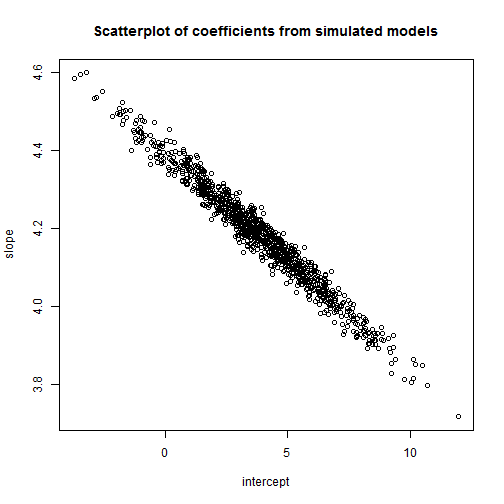We see that the variability of the slope estimates is pretty small (3.8 – 4.6), while the intercept estimates vary widely (from below 0 to 10). This jives with our original model (see output above) where the slope coefficient was signficant with a small standard error while the intercept was not significant and had a relatively large standard error.

Next we create kernel density plots of our coefficient estimates. In addition I add a vertical line to represent the mean of the 1000 coefficient estimates and add the mean itself to the plot using the text() function. I use the difference in the range of the axes to allow me to automatically determine suitable coordinates.

d1 <- density(modSim$coefficients[2, ]) d2 <- density(modSim$coefficients[1, ])
# slope
plot(d1, main = "distribution of slope coefficients")
abline(v = mean(modSim$coefficients[2, ])) text(x = mean(modSim$coefficients[2, ]) + diff(range(d1$x)) * 0.05, y = diff(range(d1$y)) *
0.05, labels = round(mean(modSim$coefficients[2, ]), 2))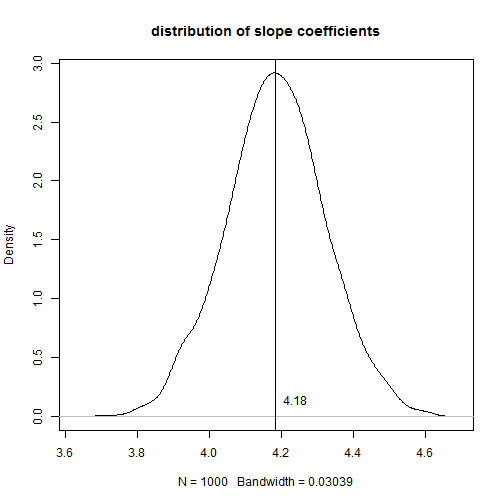# intercept plot(d2, main = "distribution of intercept coefficients") abline(v = mean(modSim$coefficients[1, ]))
text(x = mean(modSim$coefficients[1, ]) + diff(range(d2$x)) * 0.05, y = diff(range(d2$y)) * 0.05, labels = round(mean(modSim$coefficients[1, ]), 2))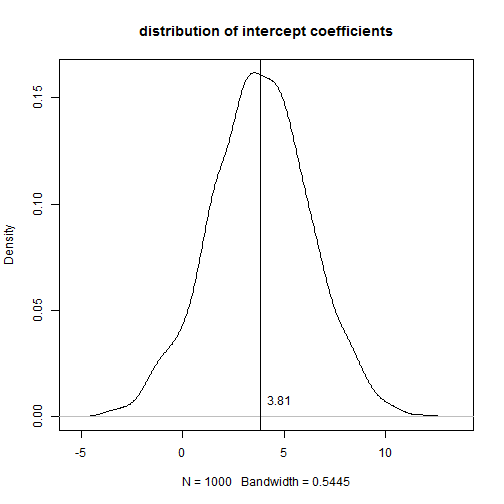If you don't compare the scale of the x-axis in the two plots the coefficients appear to have similar variability. Perhaps a better way to do it is to put both plots in one window and set the limits to the x-axis for both plots to be the range of the estimated intercept coefficients.

par(mfrow = c(2, 1))
# slope
plot(d1, main = "distribution of slope coefficients", xlim = range(d2$x)) abline(v = mean(modSim$coefficients[2, ]))
text(x = mean(modSim$coefficients[2, ]) + diff(range(d2$x)) * 0.05, y = diff(range(d1$y)) * 0.05, labels = round(mean(modSim$coefficients[2, ]), 2))
# intercept
plot(d2, main = "distribution of intercept coefficients", xlim = range(d2$x)) abline(v = mean(modSim$coefficients[1, ]))
text(x = mean(modSim$coefficients[1, ]) + diff(range(d2$x)) * 0.05, y = diff(range(d2$y)) * 0.05, labels = round(mean(modSim$coefficients[1, ]), 2))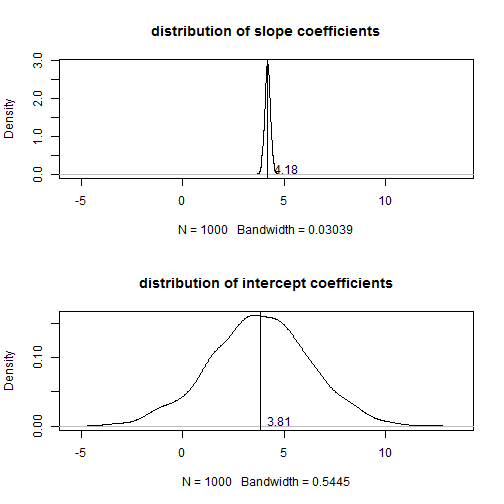par(mfrow = c(1, 1))

Now we see just how uncertain our intercept estimate is compared to the slope estimate.

Finally, why don't we go ahead and plot all 1000 fitted lines over the original data along with our original fitted line and the traditional 95% confidence band we get using our original model.

plot(x, y, col = "red", pch = 19)
abline(mod)  # original fitted line
apply(coef(modSim), 2, abline, col = "gray", lty = 3)
# predicted responses from original model
modOrig <- predict(mod, interval = "confidence")
lines(x, modOrig[, "lwr"], col = "blue")
lines(x, modOrig[, "upr"], col = "blue")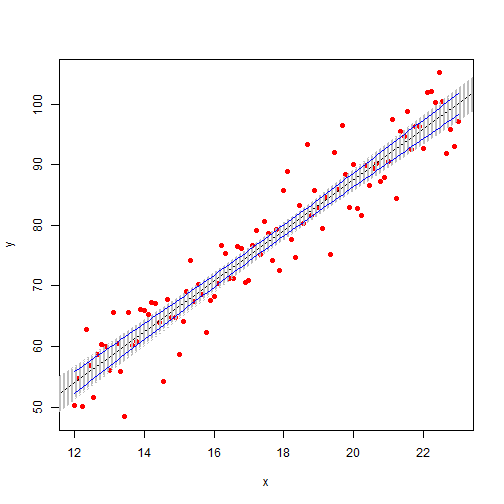The plotting of the 1000 lines essentially provides us a confidence band for the original fitted line. We see the blue lines are slightly closer to the fitted line than the band produced by our simulated models. That's because the blue lines represent the 95% confidence band. In other words, we would expect 95% of our 1000 estimated lines to fit within those blue lines. The gray lines we see outside of the blue lines are those 5% that don't fall within the 95% confidence band.

Getting started with GitHub Gist in WordPress

I’ve decided it’s time to use GitHub Gist for posting code snippets in my blog. My original motivation was better syntax highlighting. I was using WP Code Highlight, which isn’t bad. It’s easy to use and does what it promises. But it doesn’t add line numbers and the syntax highlighting doesn’t seem optimal for R. GitHub Gist on the other hand handles R code beautifully.

Now highlighted code is nice, but what’s really cool about Gist is that the code is actually stored in a repository. This means I can update the code without having to update my WordPress post. And it’s easier for other people to use should they ever want to use my code. And there are other benefits as well. I guess the only bad thing is that GitHub has my code, which would be bad if they went belly-up. I’m pretty sure that won’t happen though, because I don’t want to think about it.

So here’s how you use GitHub Gist with WordPress to host R code. First create an account on GitHub and go to GitHub Gist. Where it says “Name of file”, type the name of your file and add “.r” to the end. Next select R from the language pulldown, though Gist will probably select it for you if you typed a file name ending in “.r”. Now copy and paste your code into the editor and click “create public gist”. You’re done with GitbHub

To make this block of code appear in your WordPress post, copy the code in the “Embed this gist” field and paste into your post. (Make sure you’re in the Text editor instead of the Visual editor). It would be really nice if that was the end of it, but it’s not. WordPress strips out the embed code and nothing happens. The workaround is to add a little plug-in called Raw HTML. As the description says, “This plugin adds a set of shortcodes that you can use to “protect” specific parts of your post and prevent WP from messing with them.” Awesome, just what we need. So the final step is to surround the Gist embed code with [raw]…[/raw] shortcode. (A tip of my hat to this site for telling me about Raw HTML.

Let’s try this out. Here’s some code that samples 30 values from a N(15,2) distribution, calculates a 95% confidence interval, and plots the interval as a line. It does this 40 times and colors the CI’s that don’t capture 15 red.

To make that appear in my post, I added the following:

[raw]
<script src=”https://gist.github.com/clayford/7842136.js”></script>
[/raw]